Concept Checks: EVA

I. EVA and NPV: The Parallels

Economic value added and net present value are closely related concepts. To see the parallels, examine the following derivation:

NPVj =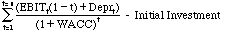This is the standard equation for the net present value of a project with a life of n years, with no salvage value at the end of the project. Note that the initial investment can be written as follows:

Initial Investment =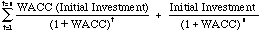Note that all we are saying is that the initial investment, if invested to earn the cost of capital, with the entire investment salvaged at the end of the project life, would be worth itself.

NPVj =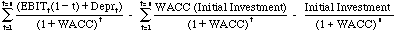=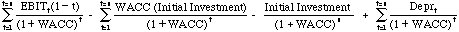Assume that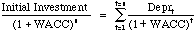:

EVA assumes that the present value of depreciation covers the present value of capital invested, i.e, it is a return of capital.

Now define ROC = EBIT (1-t) / Initial Investment

The earnings before interest and taxes are assumed to measure true earnings on the project and should not be contaminated by capital charges (such as leases) or expenditures whose benefits accrue to future projects (such as R & D). Then the NPV equation can be written as follows: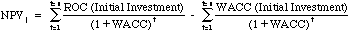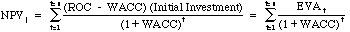2. Does increasing EVA increase firm value?

It would seem to be obvious that increasing EVA should increase firm value. This does not hold, however. The value of a firm can be written as:

Value of Firm = Capital Invested + PV of EVA from existing projects + PV of EVA from future projects

Increasing EVA in a specific year, even above expected values, may still result in a lower firm value, if

1. The increase in current year EVA is accomplished by reducing EVA in future years. Thus, the gain in EVA from the current year is more than offset by the loss in present value of future EVA. This can be called the "stealing from future growth" sceanrio.
2. The increase in EVA may be accomplished with a concurrent increase in risk and cost of capital. The present value of EVA may therefore decrease even though EVA might be higher.

The connection between EVA and stock prices is even more tenous, since the stock price reflects expected EVA. To the extent that the increase in EVA is less than expectations, you can end up with a higher EVA and lower stock prices.

Is EVA a good investment tool?

The studies that have looked at EVA have generally found little or no correlation between absolute EVA and investment returns. Richard Bernstein at Merrill lynch (Publication dated 12/19/97) looked at the returns on buying the stocks in the top decile of the S&P 500 in terms of absolute EVA and found that the portfolio earned 0.2% less than the S&P 500. Similarly, there seems to be little or no correlation between increases in EVA and investment returns. Richard Bernstein (3/2/98) found that buying the top 50 stocks in the S&P 500, based upon increases in EVA, and found that strategy earns 0.3% less than the S&P 500. Given that stock prices reflect expected EVA, neither finding should be surprising.

To use EVA in investment analysis, it seems to me that we need a good model for expected EVA changes, and analysts would then need to work on figuring out which firms will deliver changes in EVA which deviate from these expectations. Those firms which are expected to deliver better EVA than expected would be the good investments. This is analogous to what we do with earnings per share, where firms’ performance is judged against expectations.